## Friday, July 14, 2017

### 9 - Arduino: Shift Register

ARDUINO: SHIFT REGISTER

- Layout for this exercise: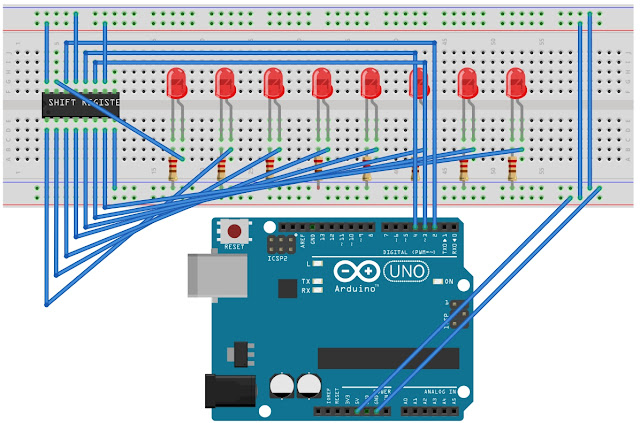1 - Introduction

- The 74HC595 Shift Register (SR) used in this exercise is an Integrated Circuit (IC) with 8 digital outputs that can be controlled with just 3 digital pins of the Arduino. To increase the number of digital outputs multiple SR could be daisy-chained, allowing unlimited number of outputs with the same 3 digital pins of the Arduino.

- Pinning of the 74HC595 Shift Register:- In this exercise two examples are explained using a Shift Register chip connected with an Arduino board.

2- Stepping up and down 8 red LEDs

- The goal of this fist exercise is to design a lighting sequence of 8 red LEDs from first to last and in reverse order.

- Arduino sketch: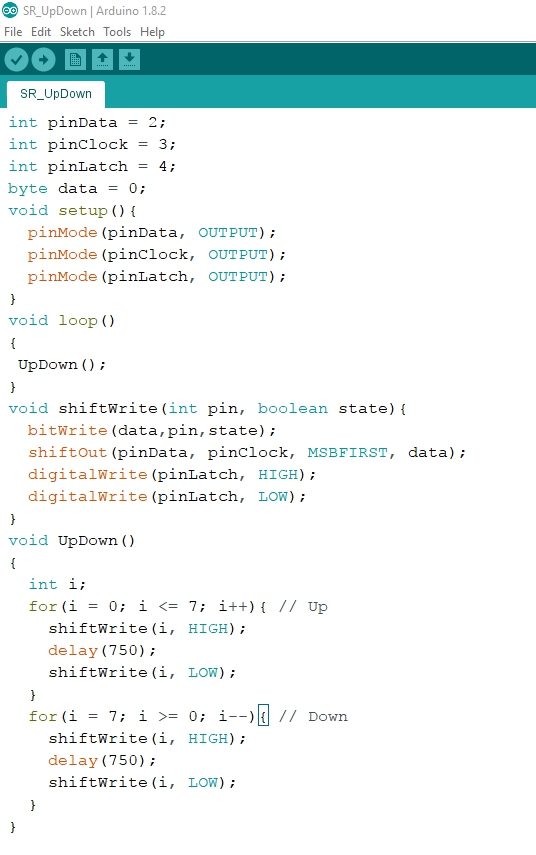- Let's analyze the code.

- The SR uses the SPI (Serial Peripheral Interface) serial connection with 3 pins:- A global variable is used to send data to the SR: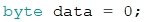- The 3 pins are set in output mode:- The loop includes the function UpDown():- The Serial Peripheral Interface (SPI) has a clock line that controls the speed of the data transfer. shiftIn() and shiftOut() are commands used to access parts that use the SPI interface.

- shiftOut() shifts out a byte of data one bit at a time, starting from either the most (in this case, MSBFIRST) or least significant bit. Each bit is written in turn to a data pin, after which a clock pin is pulsed (HIGH and LOW) to indicate that the bit is available.

- The shiftWrite() function that allows to put the SR outputs into HIGH or LOW state is composed of this subfunctions:

• bitWrite() makes individual bits of "data" (a byte) on/off,  where "pin" is the SR output from 0 to 7 and "state" is either HIGH or LOW,
•  shiftOut() shifts out a byte of data one bit at a time, starting from either the most (in this case MSBFIRST) or least significant bit. Each bit is written in turn to a data pin, after which a clock pin is pulsed (HIGH and LOW) to indicate that the bit is available.
• Toggling the pinLatch the shift register makes appear the data to the outputs, activating the high to low transition.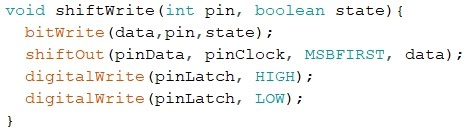- The function UpDown() defines how the 8 red LEDs are lighted one after the other in two consecutive sequences (up and down), using the shiftWrite function for each LED (from 0 to 7) and a delay of 750 milliseconds: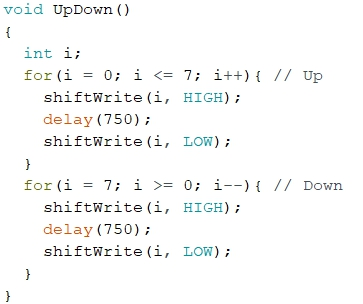- Testing the circuit and the code, the sequence of 8 LEDs light consecutively stepping up and down:

3 - Binary counter

- The goal of this exercise is to display the 0 to 255 binary bit patterns using the 8 red LEDs.

0 =     00000000
1 =     00000001
2 =     00000010
3 =     00000011
4 =     00000100
5 =     00000101
.........................
..........................

253 = 11111101
254 = 11111110
255 = 11111111

- The Arduino sketch:- Let's analyze the code.

- The first part is identical to the previous exercise, so we will go directly to the section related with the function BinaryNumbers().

- BinaryNumbers() function is called into the loop():- The function BynaryNumbers() increases every 1 second the value of the data, and writes out (shifts out, better said) that data to the 8 red LEDs: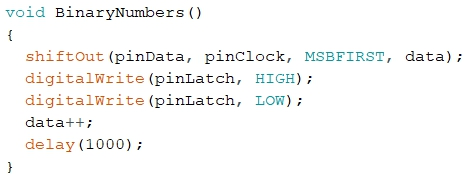- Testing the circuit and the code, the 8 red LEDs show the numbers from 0 to 255 in a binary representation :